Question

# Q11 (20pts.): For the circuit shown below Derive the transfer function, H(s) assuming the OPAMP's transfer...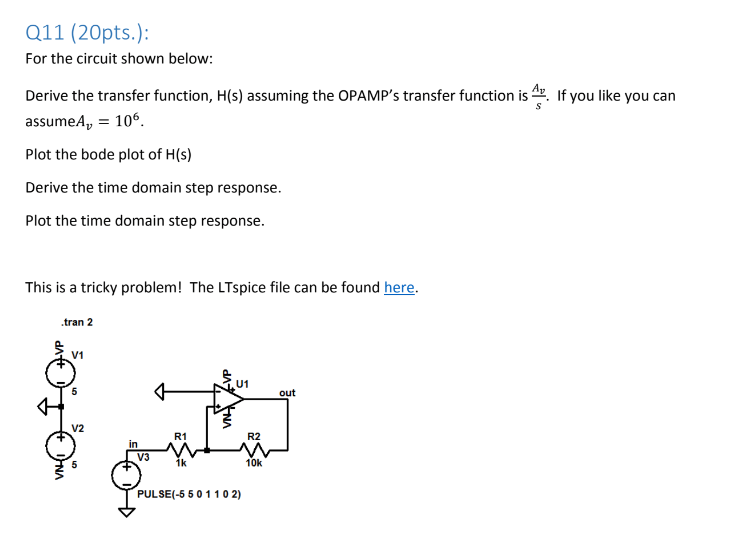Q11 (20pts.): For the circuit shown below Derive the transfer function, H(s) assuming the OPAMP's transfer function is. If you like you can assumeA 106 Plot the bode plot of H(s) Derive the time domain step response. Plot the time domain step response This is a tricky problem! The LTspice file can be found here. .tran 2 V1 U1 out V2 R1 R2 in V3 1k 10k PULSE(-5 50 1102)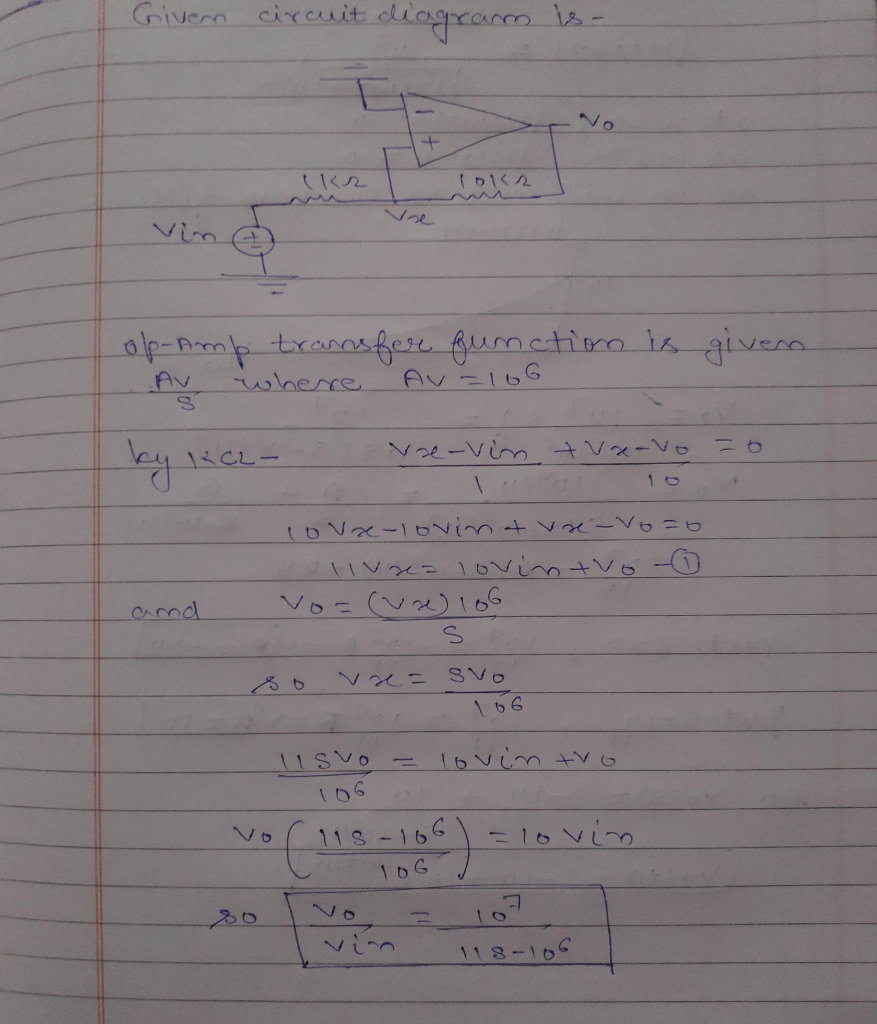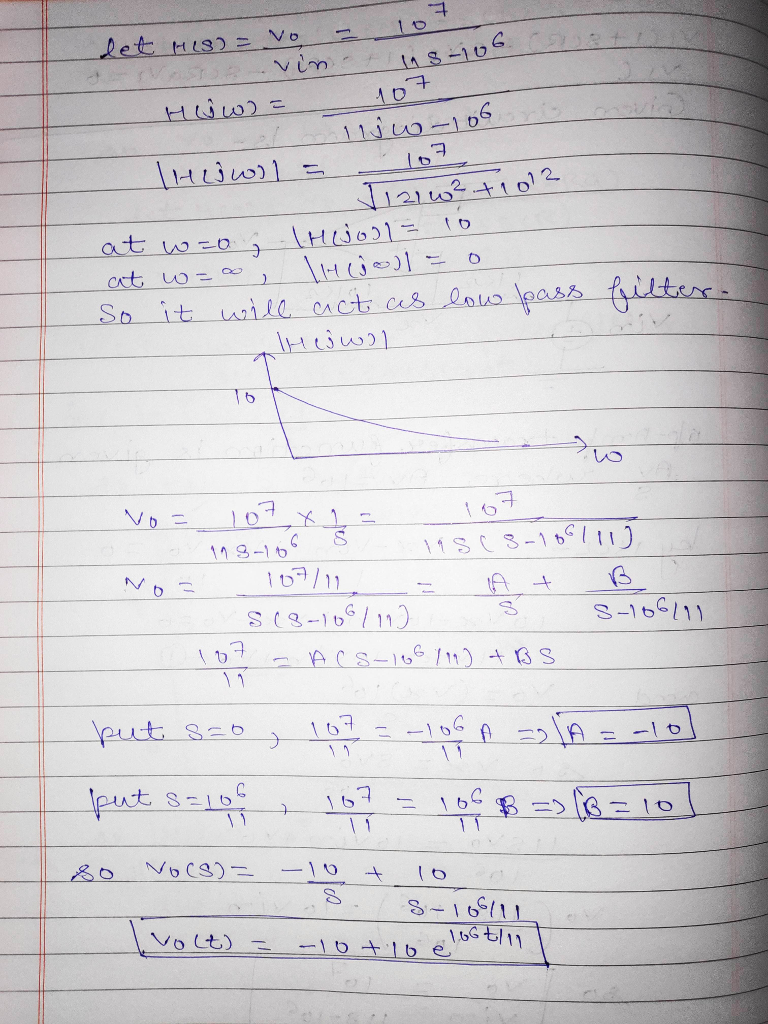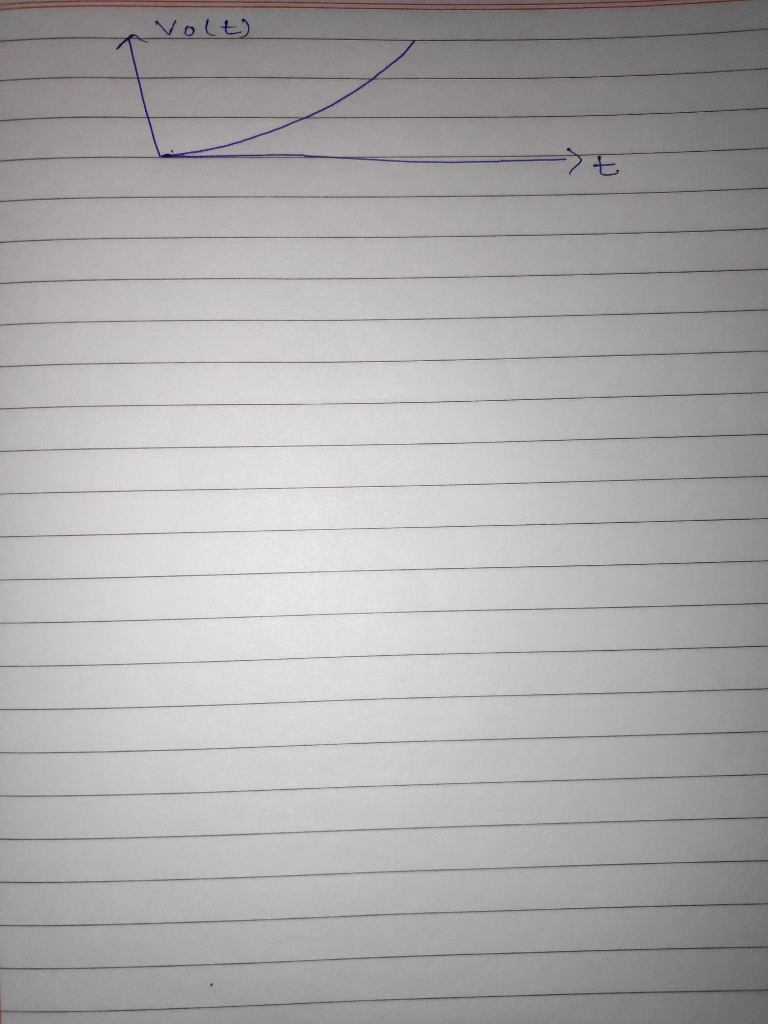#### Earn Coins

Coins can be redeemed for fabulous gifts.

Similar Homework Help Questions
• ### QUESTION #2 PLEASE 1. Derive the transfer function for the circuit shown below. Plot H(s) versus...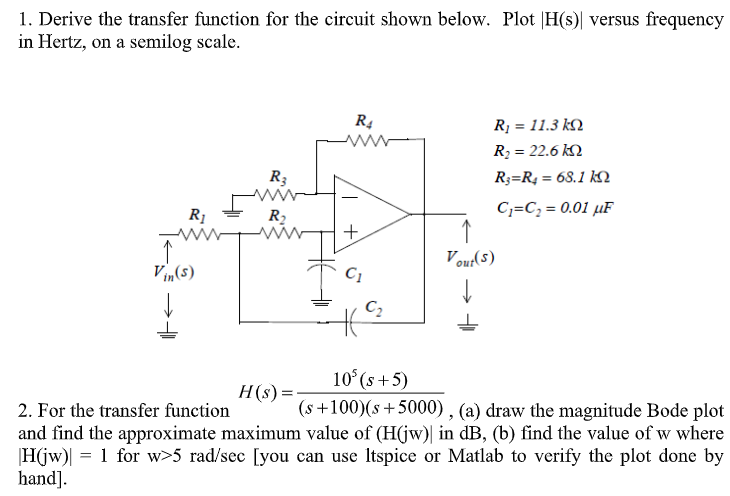QUESTION #2 PLEASE 1. Derive the transfer function for the circuit shown below. Plot H(s) versus frequency in Hertz, on a semilog scale. Ri 11.3 k Ri 22.6 k R R = 68.1 kN R3 C C 0.01 uF R2 Vout(s) Vin(s) C2 10 (s+5) H(s) = (s+100)(s5000) , (a) draw the magnitude Bode plot 2. For the transfer function and find the approximate maximum value of (H(jw) in dB, (b) find the value of w where 1 for w>5...

• ### Question 8: 1. Redraw the circuit shown below with the s domain equivalent models, where V1(t)...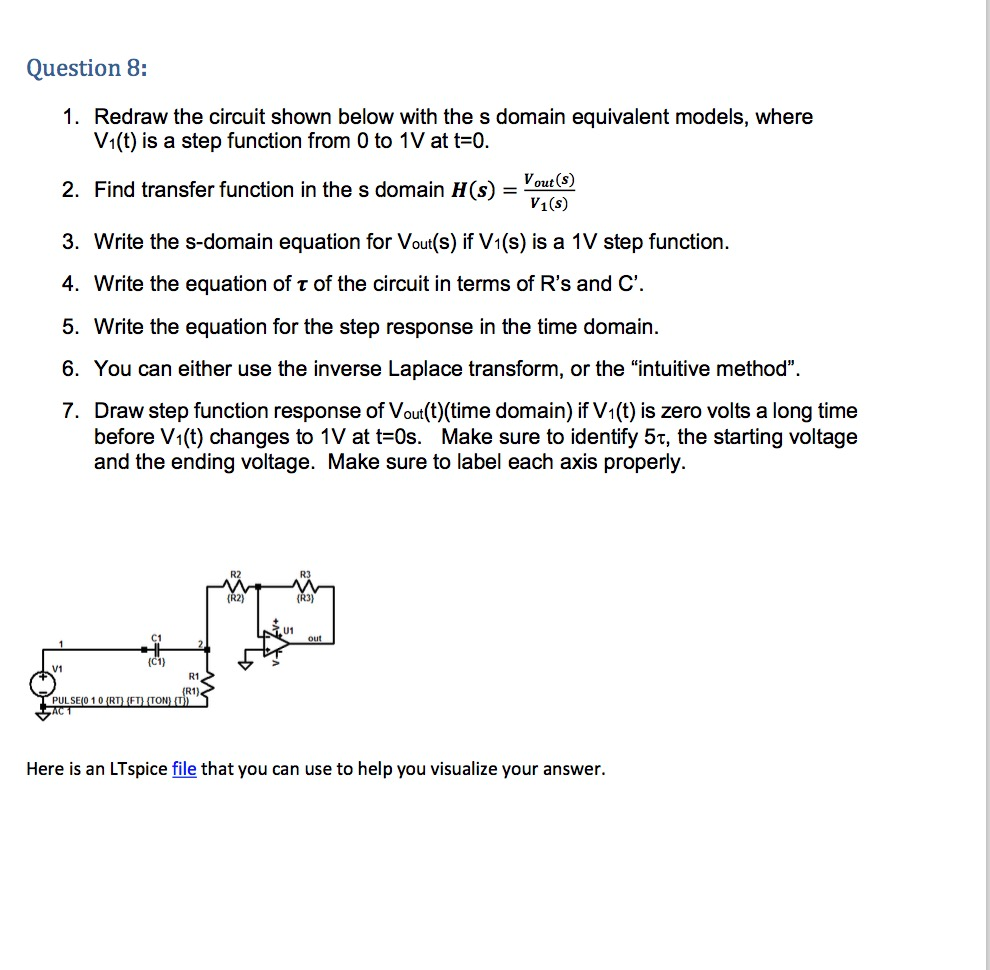Question 8: 1. Redraw the circuit shown below with the s domain equivalent models, where V1(t) is a step function from 0 to 1V at t-0 2. Find transfer function in the s domain H( 3. Write the s-domain equation for Vout(s) if V1(s) is a 1V step function. 4. Write the equation of r of the circuit in terms of R's and C 5. Write the equation for the step response in the time domain. 6. You can either...

• ### Clear Steps Please ! Q7. Derive the transfer function for circuit shown below: 0.02uF 20k V3...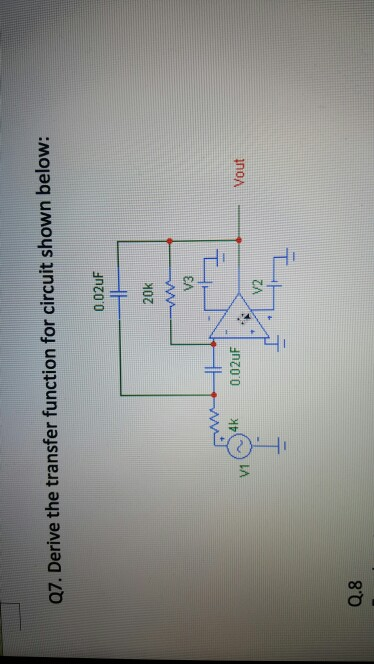Clear Steps Please ! Q7. Derive the transfer function for circuit shown below: 0.02uF 20k V3 4k 0.02uF Vout V1 V2 Q.8

• ### Find the transfer function H(s) for the circuit below. Make s=jw and plot the Bode plot...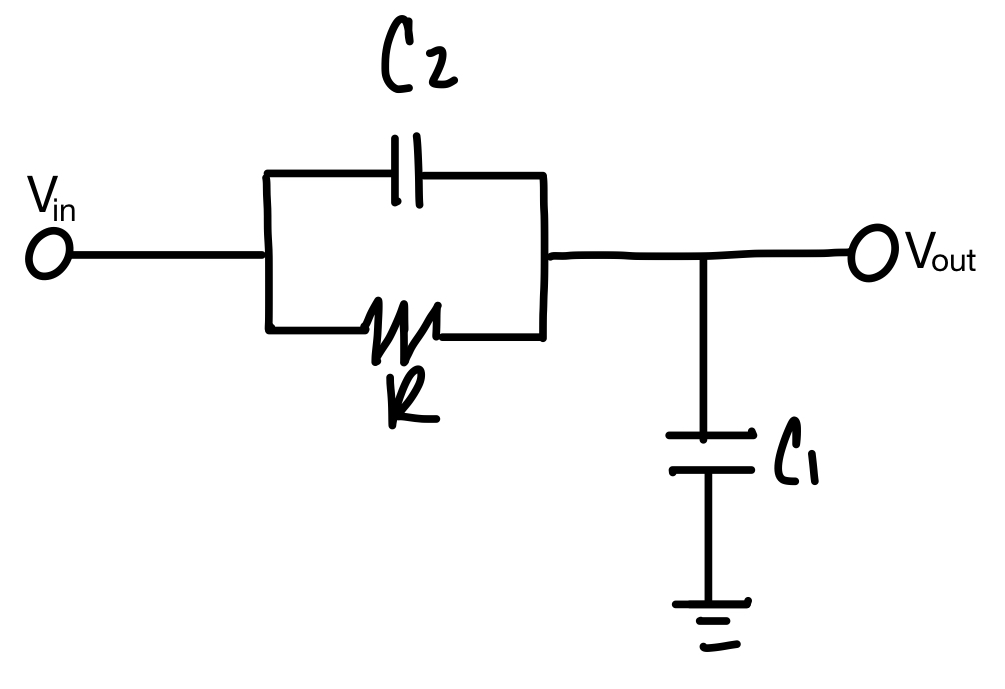Find the transfer function H(s) for the circuit below. Make s=jw and plot the Bode plot (magnitude and phase response) for the transfer function. C = out 페

• ### Please answer all parts thank you 6. [15 POINTS] Consider a passive low-pass filter as shown...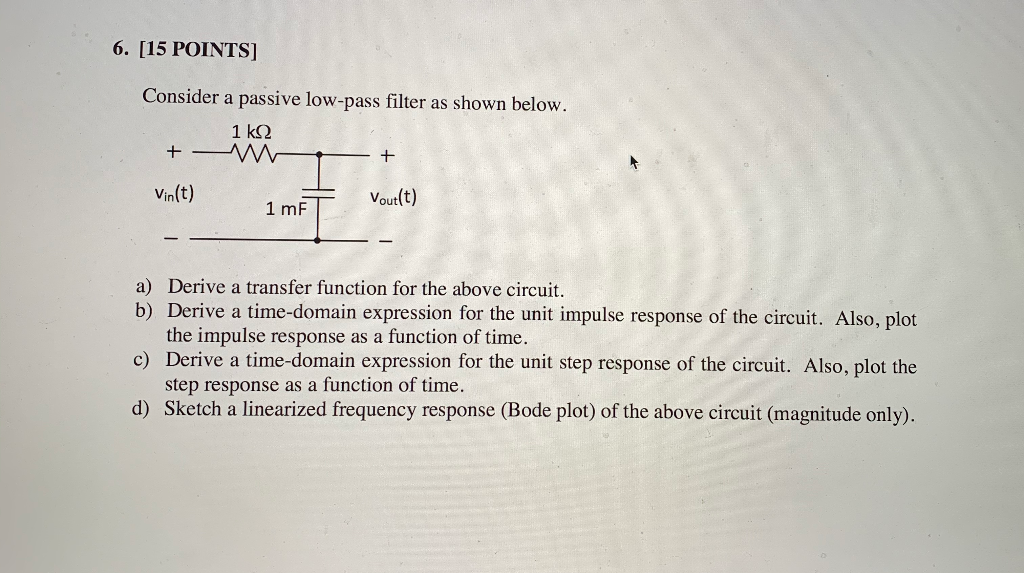Please answer all parts thank you 6. [15 POINTS] Consider a passive low-pass filter as shown below. 1k2 + - m - + Vin(t) 1 mF Vout(t) a) Derive a transfer function for the above circuit. b) Derive a time-domain expression for the unit impulse response of the circuit. Also, plot the impulse response as a function of time. c) Derive a time-domain expression for the unit step response of the circuit. Also, plot the step response as a function...

• ### Problem 1: (Time Response) Derive the transfer function (s) of the electrical circuit shown below. Then...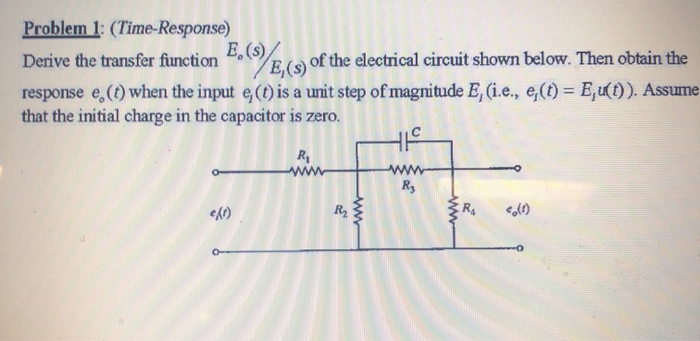Problem 1: (Time Response) Derive the transfer function (s) of the electrical circuit shown below. Then obtain the response e (0) when the input (1) is a unit step of magnitude E, (i.e., e(t) = E, (t)). Assume that the initial charge in the capacitor is zero. e/o)

• ### For the circuit shown below (1) Please derive an expression for the transfer function H(jco)- Vout/Vin....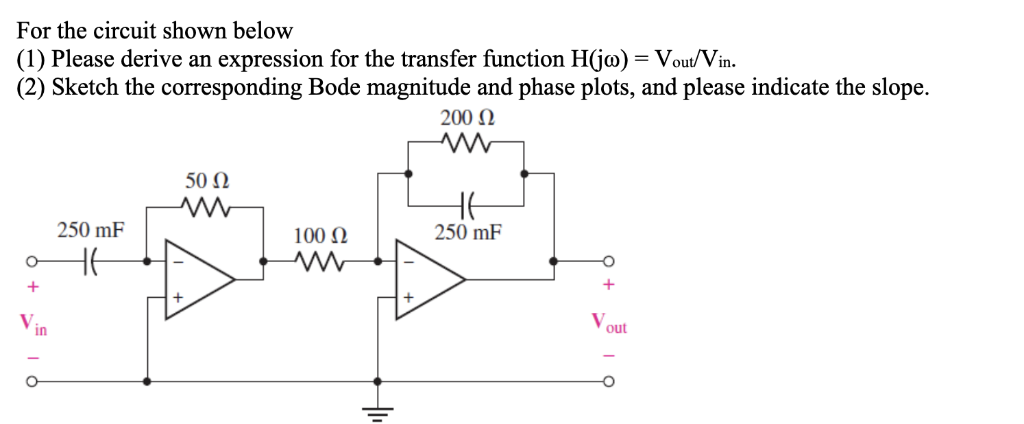For the circuit shown below (1) Please derive an expression for the transfer function H(jco)- Vout/Vin. (2) Sketch the corresponding Bode magnitude and phase plots, and please indicate the slope. 50Ω 250 mF 100 Ω 250 mF in out

• ### Pre-Laboratory Task 4: Derive an expression for the magnitude of the transfer function, H(Go)Vout(jo)/Wn(j, and the phase of the transfer function LH (ja) for the LCR circuit in Figure 4. Plot H(ja)l...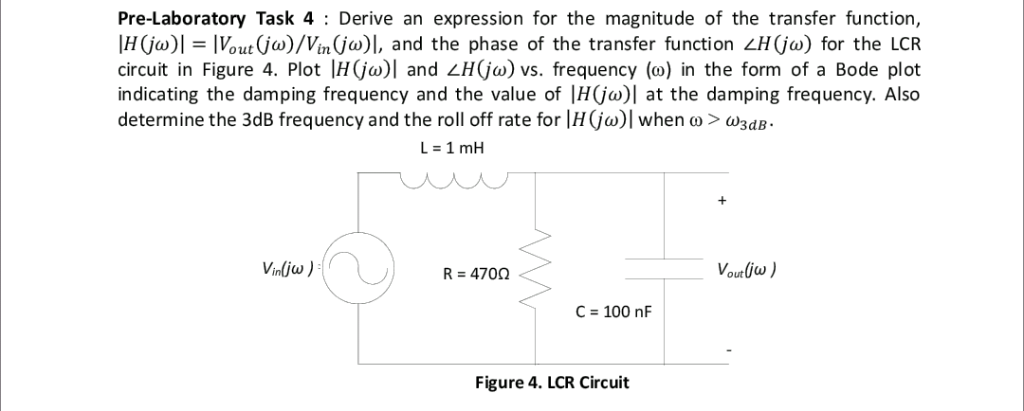Pre-Laboratory Task 4: Derive an expression for the magnitude of the transfer function, H(Go)Vout(jo)/Wn(j, and the phase of the transfer function LH (ja) for the LCR circuit in Figure 4. Plot H(ja)l and H(jo) vs. frequency (o) in the form of a Bode plot indicating the damping frequency and the value of |H(jo)| at the damping frequency. Also determine the 3dB frequency and the roll off rate for Ir(ja)1 when ω > ω3dB. Vounlius R 470Ω C 100 nF Figure...

• ### Consider the system shown as below. Draw a Bode diagram of the open-loop transfer function G(s).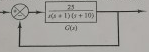1 Consider the system shown as below. Draw a Bode diagram of the open-loop transfer function G(s). Determine the phase margin, gain-crossover frequency, gain margin and phase-crossover frequency, (Sketch the bode diagram by hand) 2 Consider the system shown as below. Use MATLAB to draw a bode diagram of the open-loop transfer function G(s). Show the gain-crossover frequency and phase-crossover frequency in the Bode diagram and determine the phase margin and gain margin. 3. Consider the system shown as below. Design a...

• ### singal and system QUESTION 5 [20 marks] Given transfer function of a networks H(s) transfer function...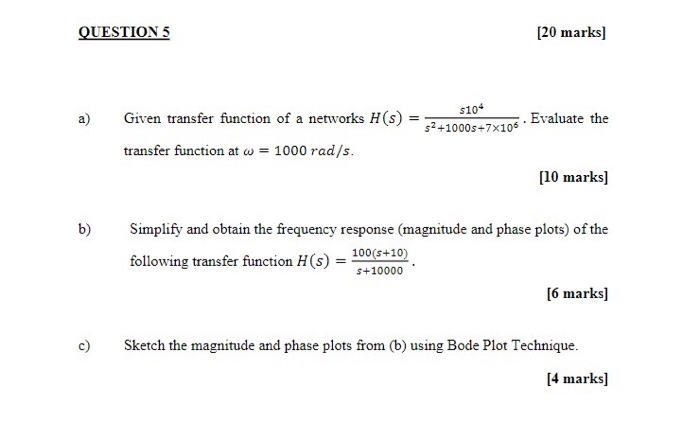singal and system QUESTION 5 [20 marks] Given transfer function of a networks H(s) transfer function at w = 1000 rad/s. \$10+ 52 +10005+7x106 - Evaluate the [10 marks) b) Simplify and obtain the frequency response (magnitude and phase plots) of the 100(5+10) following transfer function H(s) s+10000 [6 marks] Sketch the magnitude and phase plots from (b) using Bode Plot Technique. [4 marks]